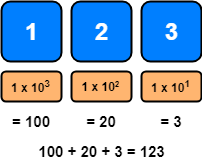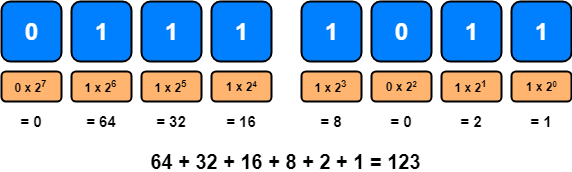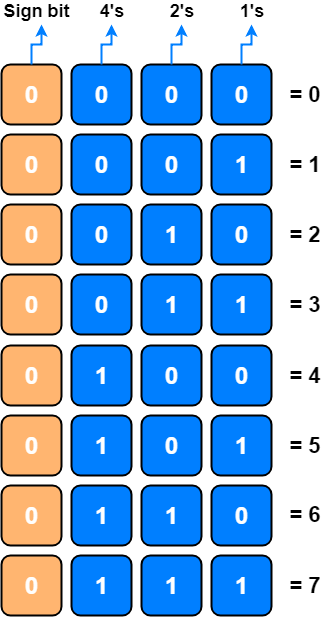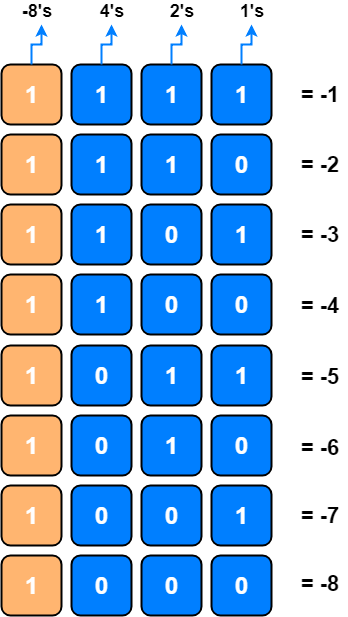# Binary Computation and Bitwise Operators

If binary code is something only computers can understand, why should you learn about it?

As developers, we have our user friendly programming languages to give instructions to computers but they are still translated into binary code for computers to be able to interpret them and run your programs.

Binary code is the most fundamental concept underlying programming and Computer Science. But, how does binary work then? How can a complex computer program consist of only 1’s and 0’s?

## How does the binary number system works?

To be able to understand the binary number system, we need to take a closer look to the decimal number system which we learn in elementary school and still using everyday. Many numeral systems of ancient civilisations use ten and its powers for representing numbers, probably because there are ten fingers on two hands and people started counting by using their fingers.1

In the decimal system, each digit in a certain number represents the 1’s, the 10’s, the 100’s, and so on, starting from the right hand side.So, with the number 123, for example, we have a 3 representing the 1’s, a 2 representing the 10’s, and finally a 1 to represent the 100’s and total of them are 123.

We are starting from 10 to the power of 0 and increasing the powers while moving to left 1, 2, 3 and so on.

In the binary system, instead of using powers of 10, we use powers of 2.In the binary system, each digit in a certain number represents 1’s, 2’s, 4’s, 8’s, 16’s, 32’s, 64’s, 128’s, 256’s and so on, starting from the right hand side.

Computers use a method called Two’s Complement 2 to represent negative numbers. Also this method can be more effective when performing mathematical operations like adding and subtracting.

In this method, the bit at the far left side of the bit pattern is the most significant bit or MSB is used to indicate positive or negative numbers.

Positive numbers always start with a 0 and the remaining bits are used to store the actual size of the number.

Four-bit, positive, two’s complement numbers would be 0000 = 0, 0001 = 1, up to 0111 = 7. The smallest positive number is the smallest binary value.Negative numbers always start with a 1 and the remaining bits are used to store the actual size of the number. The biggest negative number is the largest binary value. (e.g. 1111 is -1)## Steps to convert a positive binary number to its negative value

Following these steps will convert a positive binary number to its negative value by using Two’s Complement for negative numbers method.

1. Find the positive binary value for the negative number you want to represent.
2. Add a 0 to the front of the number, to indicate that it is positive.
3. Invert or find the complement of each bit in the number.
4. Add 1 to this number.

Ben Eater is explaining these steps very clearly in his YouTube Channel.

Even though we do need a few more digits than we did with the decimal system, the binary system is just as good as the decimal system for displaying numbers.

Well, in fact, we are not limited to numbers, different types of information can also be represented in binary code, too!

To be able to represent text in binary code, we can use simple numbers to represent the different letters in the alphabet. So, A could be 1, B could be 2, and so on.

Also, images and graphics displayed on your screen consists of pixels and each pixel in an image has a numerical value that determines the color it should display. This means that, we can represent images and graphics with binary code.

## Bitwise Operators

Working with primitive data types like bytes, booleans, ints, floats, doubles or with data structures is normal for a programmer. Sometimes in Computer Science, you need to go beyond this to a more deeper level where you need to understand the importance of bits.

This is especially important if you are working on data compression and encryption algorithms, optimization, data correction and error detection algorithms and so on.

Lets start with basics. Followings are the bitwise operators that we can use in many programming languages.

Operator Desctiprion
& AND
| OR
^ XOR (Exclusive OR)
~ One’s Complement
« Left Shift
» Right Shift

### AND Operator

AND Operator (`&`) gives 1 only if both of the operands are 1, otherwise 0.

A B A&B
0 0 0
0 1 0
1 0 0
1 1 1
``````A = 1, B = 3, A&B = 1
``````

Proof:

``````    0001   (A)
0011   (B)
& -------
0001   (A&B) = 1
``````

### OR Operator

OR Operator (`|`) gives 0 only if both of the operands are 0, otherwise 1.

A B A|B
0 0 0
0 1 1
1 0 1
1 1 1
``````A = 1, B = 2, A|B = 3
``````

Proof:

``````    0001   (A)
0010   (B)
| -------
0011   (A|B) = 3
``````

### XOR Operator

XOR Operator (`^`) gives 1 for odd numbers of 1s, otherwise 0.

A B A^B
0 0 0
0 1 1
1 0 1
1 1 0
``````A = 2, B = 7, A^B = 5
``````

Proof:

``````    0010   (A)
0111   (B)
^ -------
0101   (A^B) = 5
``````

### One’s Complement Operator

One’s Complement Operator (`~`) is an unary operator and converts 0s to 1s and 1s to 0s.

``````X = 2, ~X = -3
``````

Proof:

32-bit

`````` 2 = 0000 0000 0000 0000 0000 0000 0000 0010
~2 = 1111 1111 1111 1111 1111 1111 1111 1101 = -3
``````

### Left Shift Operator

Left Shift Operator (`<<`) shifts the bits to the left.

``````X = 4, X<<1 = 8
``````

Proof:

``````X    = 0100
X<<1 = 1000 ---> 8
``````

### Right Shift Operator

Right Shift Operator (`>>`) shifts the bits to the right.

``````X = 4, X>>2 = 1
``````

Proof:

``````X    = 0100
X>>2 = 0001 ---> 1
``````
1. Wikipedia, Origin of the Decimal Number System
2. Wikipedia, Two’s complement